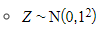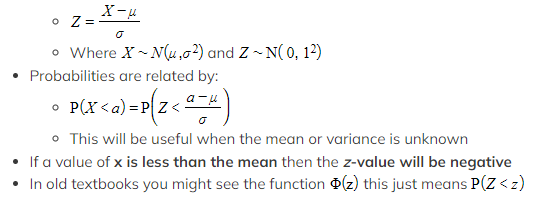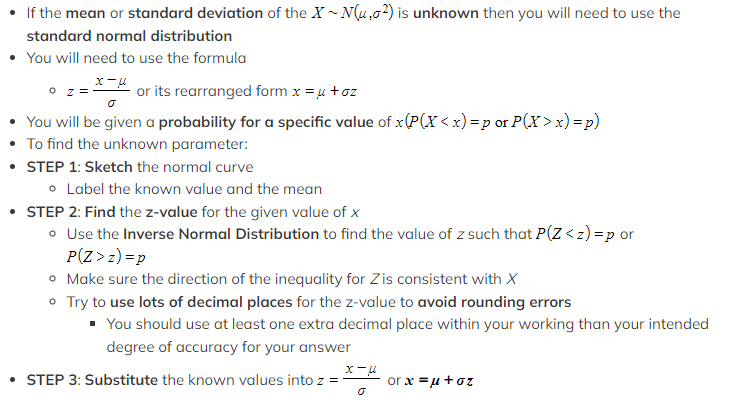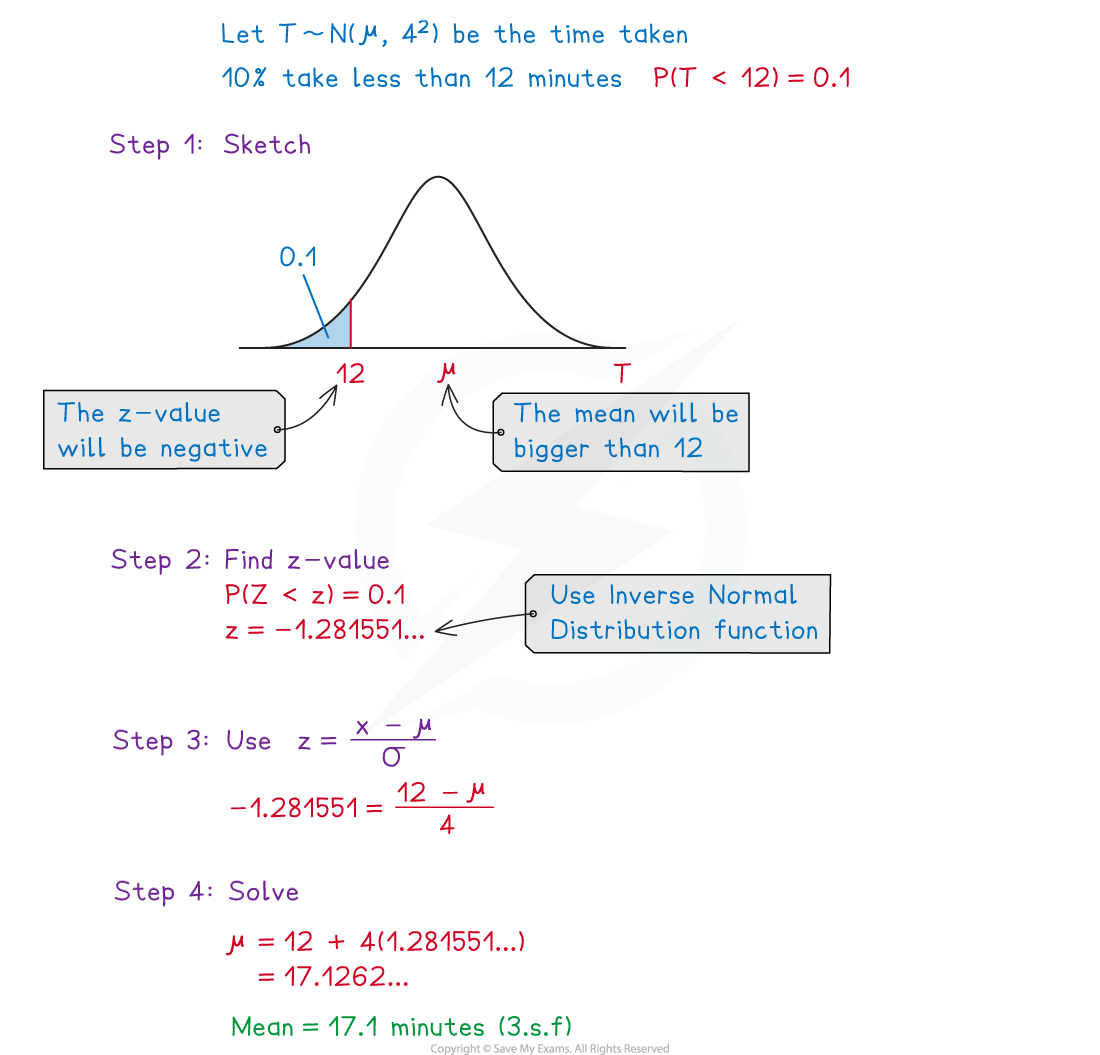# AQA A Level Maths: Statistics复习笔记4.3.3 Standard Normal Distribution

### Standard Normal Distribution

#### What is the standard normal distribution?

• The standard normal distribution is a normal distribution where the mean is 0 and the standard deviation is 1
• It is denoted by Z

####Why is the standard normal distribution important?

• Any normal distribution curve can be transformed to the standard normal distribution curve by a horizontal translation and a horizontal stretch
• Therefore we have the relationship:

###Finding Sigma and Mu

#### How do I find the mean (μ) or the standard deviation (σ) if one of them is unknown?You will be given x and one of the parameters (μ  or σ) in the question

• You will have calculated z in STEP 2
• STEP 4: Solve the equation

#### How do I find the mean (μ) and the standard deviation (σ) if both of them are unknown?

• If both of them are unknown then you will be given two probabilities for two specific values of x
• The process is the same as above
• You will now be able to calculate two z-values

####Worked Example

It is known that the times, in minutes, taken by students at a school to eat their lunch can be modelled using a normal distribution with standard deviation 4 minutes.

Given that 10% of students at the school take less than 12 minutes to eat their lunch, find the mean time taken by the students at the school.#### Exam Tip

• These questions are normally given in context so make sure you identify the key words in the question. Check whether your z-values are positive or negative and be careful with signs when rearranging.